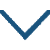早春呈水部张十八员外

• zǎo
• chūn
• chéng
• shuǐ
• zhāng
• shí
• yuán
• wài
•
• táng
•
• hán
• tiān
• jiē
• xiǎo
• rùn
•
• cǎo
• yáo
• kàn
• jìn
• què
•
• zuì
• shì
• nián
• chūn
• hǎo
• chù
•
• jué
• shèng
• yān
• liǔ
• mǎn
• huáng
•

译文

京城大道上空丝雨纷纷，它像酥油般细密而滋润，
远望草色依稀连成一片，近看时却显得稀疏零星。
这是一年中最美的季节，
远胜过绿柳满城的春末。

注释

呈：恭敬地送给。水部张十八员外：指张籍（766—830年）唐代诗人。在同族兄弟中排行第十八，曾任水部员外郎。
天街：京城街道。润如酥：细腻如酥。酥，动物的油，这里形容春雨的细腻。
最是：正是。处：时。
绝胜：远远胜过。皇都：帝都，这里指长安。

赏析

这是一首描写和赞美早春美景的七言绝句。第一句写初春的小雨，以“润如酥”来形容它的细滑润泽，十分准确地写出了它的特点，遣词用句十分优美。与杜甫的“好雨知时节，当春乃发生。随风潜入夜，润物细无声”有异曲同工之妙。
第二句紧承首句，写草沾雨后的景色。以远看似青 ，近看却无 ，描画出了初春小草沾雨后的朦胧景象。可与王维的"青霭入看无"、"山色有无中"相媲美。
三、四两句对初春景色大加赞美：最是一年春好处，绝胜烟柳满皇都。写春景的诗，在唐诗中，多取明媚的晚春，这首诗却取早春咏叹，认为早春比晚春景色优胜，别出新意。
这首诗咏早春，能摄早春之魂，给读者以无穷的美感趣味，甚至是绘画所不能及的。诗人没有彩笔，但他用诗的语言描绘出极难描摹的色彩——一种淡素的、似有却无的色彩。如果没有锐利深细的观察力和高超的诗笔，便不可能把早春的自然美提炼为艺术美。• xià
• jué
•
• sòng
•
• qīng
• zhào
• shēng
• dāng
• zuò
• rén
• jié
•
• wéi
• guǐ
• xióng
•
• zhì
• jīn
• xiàng
•
• kěn
• guò
• jiāng
• dōng
•• fēng
•
• táng
•
• luó
• yǐn
• lùn
• píng
• shān
• jiān
•
• xiàn
• fēng
• guāng
• jìn
• bèi
• zhàn
•
• cǎi
• bǎi
• huā
• chéng
• hòu
•
• wèi
• shuí
• xīn
• wèi
• shuí
• tián
•• shì
• ér
•
• sòng
•
• yóu
• yuán
• zhī
• wàn
• shì
• kōng
•
• dàn
• bēi
• jiàn
• jiǔ
• zhōu
• tóng
•
• wáng
• shī
• běi
• dìng
• zhōng
• yuán
•
• jiā
• wàng
• gào
• nǎi
• wēng
•• lín
• ān
•
• sòng
•
• lín
• shēng
• shān
• wài
• qīng
• shān
• lóu
• wài
• lóu
•
• 西
• shí
• xiū
•
• nuǎn
• fēng
• xūn
• yóu
• rén
• zuì
•
• zhí
• háng
• zhōu
• zuò
• biàn
• zhōu
•• guān
• shū
• yǒu
• gǎn
•
• sòng
•
• zhū
• bàn
• fāng
• táng
• jiàn
• kāi
•
• tiān
• guāng
• yún
• yǐng
• gòng
• pái
• huái
•
• wèn
• qīng
•  ?
• wèi
• yǒu
• yuán
• tóu
• huó
• shuǐ
• lái
•微信公众号